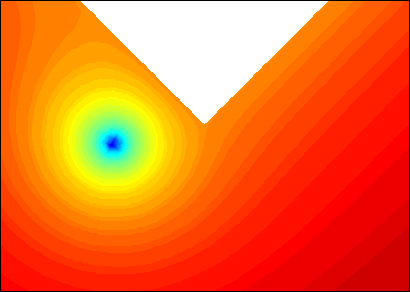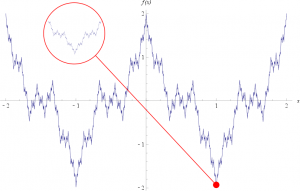# Our Favourite Functions: Part I

Because we love functions!Functions are rather important in maths.  From the humble $y=$ constant to the exotic special functions, from straight lines to never-ending spirals, from those in one variable to those that live in multidimensional worlds, they’re pretty hard to escape.  So we asked people what their favourite function is and you’ll find their contributions below.  We’d love to hear what your one is, so get in touch at contact@chalkdustmagazine.com and you can also read Part II of this blog.

### Stream Function (Pietro Servini)Source: Oliver Southwick

In incompressible fluid dynamics (where the density of the liquid or gas remains approximately constant), the stream function ($\psi$) is a very useful thing.  The two dimensional version was introduced by Lagrange in 1781 and in Cartesian coordinates satisfies $u = \partial \psi/\partial y$ and $v = – \partial \psi/\partial x$, where $u$ and $v$ are the fluid velocities in the $x$ and $y$ directions respectively.  Lines where $\psi$ is a constant are known as streamlines which, when plotted, show us the direction in which a fluid element will travel next and can result in beautiful pictures, such as this one modelling the formation of an eddy (centre in blue) as currents turn around the southern tip of Africa (in white).

### Hyperbolic Cosine (Adam Townsend)This is a weird looking function, $\cosh(x) = (e^x + e^{-x})/2$, and it has a funny name (hyperbolic cosine?!) but it turns out to be a function that you see drawn every day! If you draw this function, it looks like a U, and in fact it’s identical to the shape you get if you hang a chain between two points. It’s called a catenary (because maths needs more terms, right?) and the fact that this function turns up in other parts of maths as well (you first see it when solving certain differential equations), I think is really cool.

### Indicator Function (Rafael Prieto Curiel)

Sometimes the simplest things are the most interesting. Hence my favourite function simply counts things, but in a smart way: it tells us, from a particular set of objects, how many are at most a certain distance from a fixed point. It might sound trivial, since it only counts stuff, but its power is fantastic.  For example, it can tell us, like in the image, how many London Underground stations are at a walkable distance of 400 metres from where we are (like the function in the picture).  Or we could count the more than 100 billion stars in our galaxy, or the over 1,000 operational satellites that are orbiting the Earth.  Or tell the difference between carbon (six electrons around the nucleus of an atom) and ununpentium (115).  Me, I would love to know how many pubs are open until late and are close to my house, or how many mathematicians are currently living in London, and those numbers could be obtained just by evaluating the function wisely.

### Weierstrass Function (Anna Lambert)My favourite function has infinitely many zigzags. It’s called the Weierstrass function, and is a classic feature of a first term analysis course. It’s written
\begin{equation*}
f(x) = \sum_{n=0}^{\infty}a^n \cos(b^n \pi x)
\end{equation*}
where $0 < a < 1$, $b$ is a positive odd integer and $ab > 1+\frac{3}{2}\pi$. It might not look like much, but it was the first known example of a function that is continuous but nowhere differentiable. What does that mean? Well, a function is continuous if you can draw it without taking your pen off the paper. It is differentiable if the slope of the function varies smoothly, but it will fail to be differentiable at a point if that point is sharp. For example, a zigzag is continuous, and differentiable everywhere except for at its zigs and zags. So for a function to be nowhere differentiable, every single point on the curve must be sharp. This is an incredibly weird concept to think about. Clearly all of these sharp points cannot be visible at once, but as you zoom in, you can see more and more zigs and zags. This is just like a fractal—as you magnify, the curve looks the same and reveals even more detail.

### Hat Function (Matthew Scroggs)My favourite function is the piecewise linear hat function.
$$f(x)=\left\{ \begin{array}{cl} 0&\mathrm{if\ }x<x_{i-1}\\ \frac{x-x_{i-1}}{x_i-x_{i-1}}&\mathrm{if\ }x_{i-1}\leq x<x_i\\ \frac{x-x_{i+1}}{x_i-x_{i+1}}&\mathrm{if\ }x_i\leq x <x_{i+1}\\ 0&\mathrm{if\ }x_i\geq x_{i+1} \end{array} \right.$$

The function is zero outside the range $(x_{i-1},x_{i+1})$, one at $x_i$ and linear on the sections $(x_{i-1},x_i)$ and $(x_i,x_{i+1})$.

Partial differential equations (PDEs) are a type of equation telling us how various quantities are changing and are used to model a large variety of situations, including those in the fields of acoustics, electromagnetics and quantum mechanics. PDEs are often very hard (or even impossible) to solve, and so numerical methods that give a very good approximation to the solution are required.

One such method is the finite element method, which breaks the x-axis into lots of smaller sections and then uses functions on these sections to make the difficult PDE into a set of simultaneous equations that is easier to solve. The piecewise linear hat function is the most common function used for this method.

### Popcorn Function (Belgin Seymenoglu)My favourite function is called Thomae’s function, but it has many other weird and wonderful names, such as the raindrop function, ruler function, Stars over Babylon and my personal favourite: the popcorn function.
$$f(x)=\left\{ \begin{array}{cl} \frac{1}{q}&\mathrm{if\ }x\mathrm{\ is\ rational} \mathrm{\ and\ } x=\frac{p}{q}\quad\mathrm{\ (in\ lowest\ terms)}\\\\ 0&\mathrm{if\ }x\mathrm{\ is\ irrational} \end{array} \right.$$

What makes the popcorn function remarkable is that it is discontinuous on the rational numbers (or fractions), yet is continuous everywhere else (i.e. on the irrational numbers).

### Function Function (Matthew Wright)

Let $\mathbb{S}$ be the set of all strings of letters from the Roman alphabet. Then my favourite function is the map $F: \mathbb{S}\rightarrow \mathbb{S}$ such that
\begin{align*}
F(x)=\begin{cases} \textrm{fun}x &\textrm{ if } x=\textrm{ctions} \\
x &\textrm{ otherwise} \end{cases}
\end{align*}
Why do I like this function? Because it is the function which puts the fun in functions! (sorry…)

Think we can do better? Email us at contact@chalkdustmagazine.com with a few sentences about your favourite function and we’ll feature them in Part II of this blog in a few weeks time.